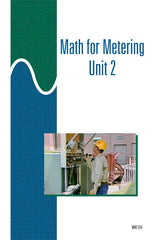#### Get the Study Guide for this videoFollow the video session-by-session with this handy book. Color illustrations throughout. \$15

Instructor Guide  available

# Math for Metering 2 - DVD

### \$ 145.00

##### Summary

This 37-minute DVD explains how sine waves can be drawn as X-Y graphs, and how they can be represented as phasors and phasor diagrams. It explains the relationships between the sides of right triangles, and how right triangles can be used to represent voltage, current, and power factor to solve metering problems.

##### Training Sessions

Basic Graph Characteristics – 7 minutes
Identifies characteristics of an X-Y graph. Demonstrates how values can be located and plotted on an X-Y graph. Describes how some of the characteristics of a basic X-Y graph are related to sine wave graphs and phasor diagrams.

Phasors and Phasor Diagrams – 11 minutes
Explains what a phasor is, identifies phasors commonly used in electrical applications, and explains how a phasor can be used to represent the magnitude and direction of a quantity. Describes or demonstrates three methods of phasor addition.

Basic Trigonometric Principles – 9 minutes
Describes the characteristics of a right triangle. Explains how the Pythagorean theorem can be used to calculate the value of one side of a right triangle, when the values of the other two sides are known. Explains how to determine the sine, cosine, and tangent of an angle.

Applying Math to Metering – 10 minutes
Explains how to: use current and voltage values to determine the power drawn by a circuit, determine the power factor for a circuit using the cosine function, use a power triangle to determine the apparent power drawn by a circuit.

Item Code: MM2-DVD